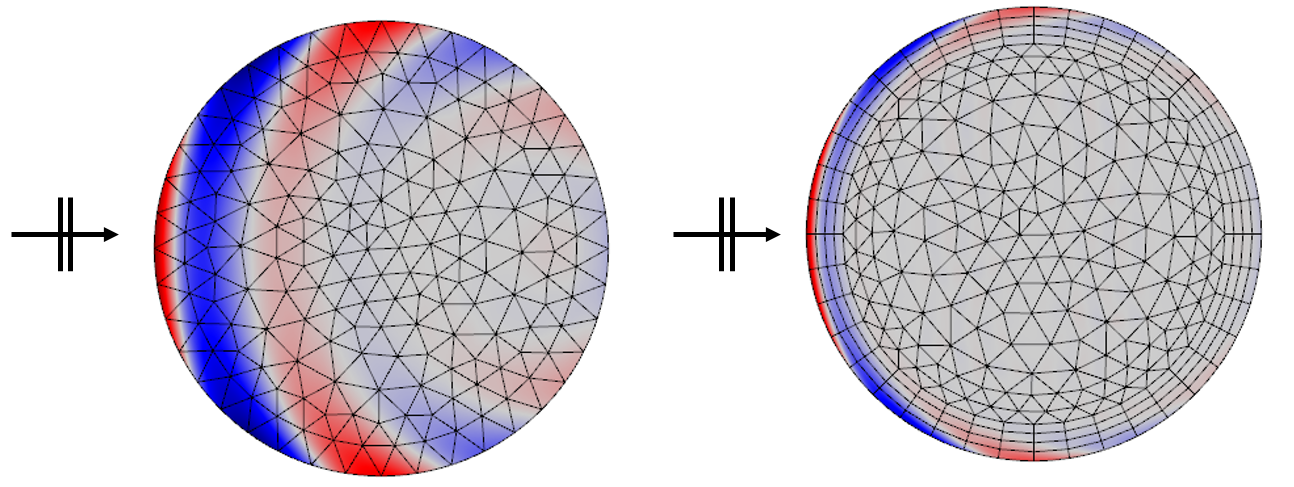# 电磁波问题中的材料建模

2015年 5月 27日

### 我们在求解哪些方程组？

\nabla \times \left( \mu_r^{-1} \nabla \times \mathbf{E} \right)-\frac{\omega^2}{c_0^2} \left( \epsilon_r -\frac{j \sigma}{\omega \epsilon_0} \right) \mathbf{E}= 0

### 电导率

\sigma = \frac{1}{\rho_0 (1 + \alpha ( T-T_{ref} )) }

RF 模块还提供了其他两个选项来计算均匀电导率：Archie 定律（用于计算充满导电流体的不导电多孔介质的等效电导率）和混合了多种材料的多孔介质模型。

Archie 定律模型常用于模拟饱含海水、原油或其他电导率要高于土壤的流体的土壤。

\sigma_{eff}=\sideset{}{^n_{i=1}}
\sum \theta_i \sigma_i

\frac{1}{\sigma_{eff}} = \sideset{}{^n_{i=1}}
\sum\frac{\theta_i}{ \sigma_i}

\sigma_{eff} = \sideset{}{^n_{i=1}}
\prod\sigma_i^{\theta_i }

### 相对介电常数

Drude-Lorentz 弥散是基于 Drude 自由电子模型和 Lorentz 振荡模型开发的材料模型。Drude 模型 (\omega_0=0) 用于金属和掺杂半导体，Lorentz 模型描述了声子模及带间跃迁等谐振现象。通过加和来结合这两个模型，将能精确描述各类固体材料。它预测了复相对介电常数随频率的变化：

\epsilon_r=\epsilon_{\infty}+\sideset{}{^M_{k=1}}
\sum\frac{f_k\omega_p^2}{\omega_{0k}^2-\omega^2+i\Gamma_k \omega}

Debye 弥散模型是 Peter Debye 基于极化弛豫时间开发的材料模型。模型主要用于极性液体。它预测了复相对介电常数随频率的变化：

\epsilon_r=\epsilon_{\infty}+\sideset{}{^M_{k=1}}
\sum\frac{\Delta \epsilon_k}{1+i\omega \tau_k}

\epsilon_r=1+\sideset{}{^M_{k=1}}
\sum\frac{B_k \lambda^2}{\lambda^2-C_k}

### 模拟与网格剖分注意事项

\delta=\left[ \operatorname{Re} \left( \sqrt{j \omega \mu_0 \mu_r (\sigma + j \omega \epsilon_0 \epsilon_r)} \right) \right] ^{-1}